## Analogy

#### Analogy

Direction: Select the related figure from the answer figures.

1. Find the question mark ? figure from answer figure.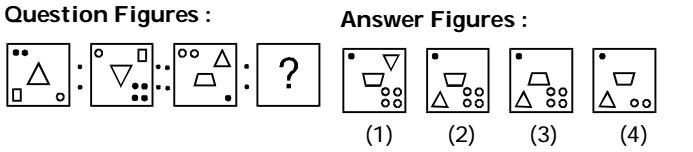1. From first figure to second figure the circles interchange position and the circles which come to the lower right corner get duplicated. The rectangle moves upward diagonally while the central design (D) is inverted.

##### Correct Option: B

From first figure to second figure the circles interchange position and the circles which come to the lower right corner get duplicated. The rectangle moves upward diagonally while the central design (D) is inverted.

1. Find the question mark ? figure from answer figure.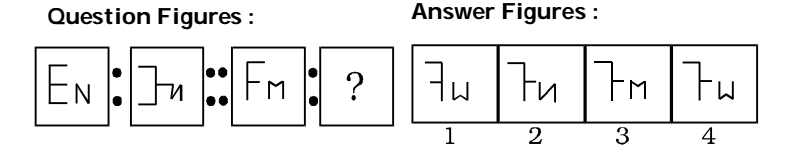1. From first figure to second figure both the designs are inverted horizontally but the middle line segment of the left design remains constant.

##### Correct Option: C

From first figure to second figure both the designs are inverted horizontally but the middle line segment of the left design remains constant.

1. Find the question mark ? figure from answer figure.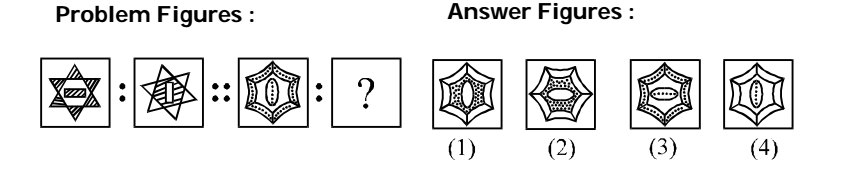1. From first figure to second shaded part becomes white and vice-versa and the central design is rotated through 90°.

##### Correct Option: B

From first figure to second shaded part becomes white and vice-versa and the central design is rotated through 90°.

1. Find the question mark ? figure from answer figure.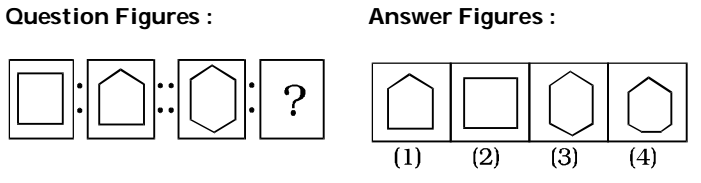1. From first figure to second figure one side is increased.

##### Correct Option: D

From first figure to second figure one side is increased.

Direction: Select the related figure from the given alternatives.

1. Find the question mark ? figure from answer figure.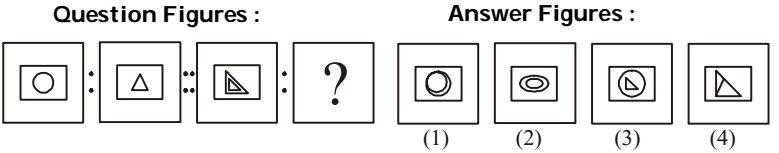1. From first figure to second figure the inner design is replaced with a new design.

##### Correct Option: B

From first figure to second figure the inner design is replaced with a new design.